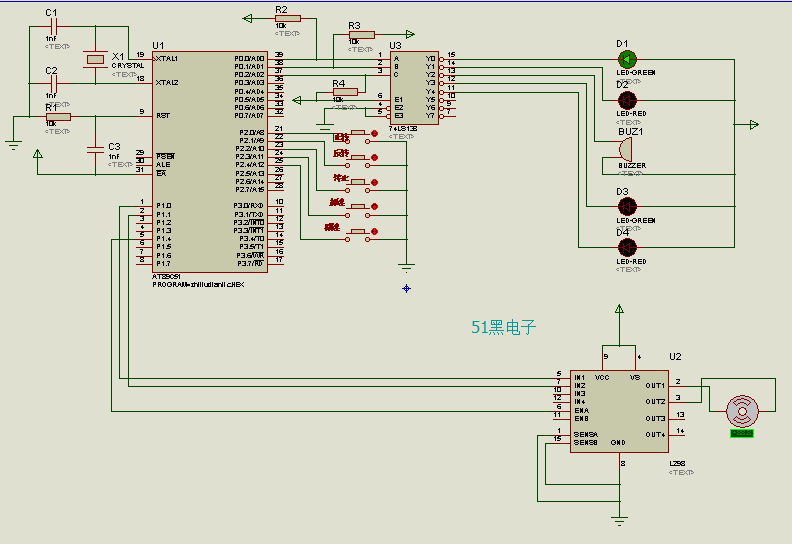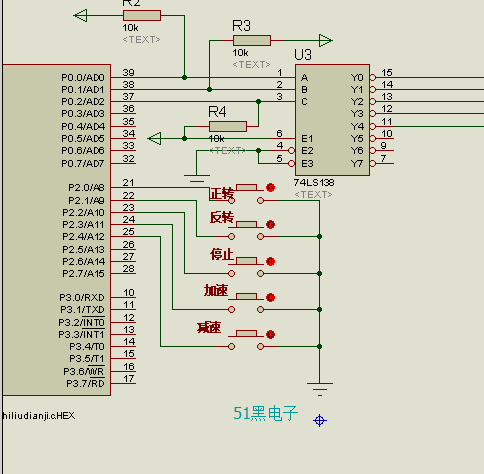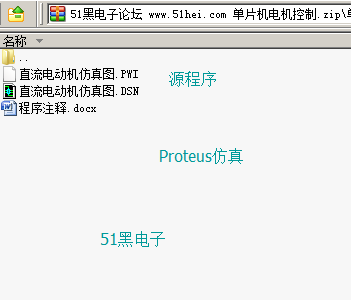##用户名 Email 自动登录 找回密码 密码 立即注册

# 单片机控制直流电机程序(注释详细)与Proteus仿真[复制链接]H昌S 发表于 2019-4-15 15:43 | 显示全部楼层 |阅读模式
 仿真基本效果1.       按下正转按钮，绿灯长亮，电机低速正转，档位为1档。2.       按下正转按钮，绿灯长亮，电机低速正转，档位为1档。3.       在未达到最高档（3档）的情况下，按下加速按钮，电机档位加一，绿灯闪烁8次，每次闪烁的时间间隔为1秒。如果达到最高档，按下加速按钮，电机档位不变。然后判断电机的转动方向，如果正转，绿灯常亮；如果反转，红灯常亮。4.       在未达到最低档位（1档）的情况下，按下减速按钮，电机档位减一，红灯闪烁8秒，每次闪烁的室间隔为1秒。如果达到最低档，按下减速按钮，电机档位不变。然后判断电机的转动方向，如果正转，绿灯常亮；如果反转，红灯常亮。5.       按下停止按钮，蜂鸣器报警，且所有LED灯灭，电机停止，并使电机档位置1档。 仿真原理图如下（proteus仿真工程文件可到本帖附件中下载）单片机源程序如下: #include #define uint unsigned int #define uchar unsigned char uchar m;               //用来标志速度档位 uint t;                           //计数标志 sbit k1=P2^0;     //正转按钮 sbit k2=P2^1;      //反转按钮   sbit k3=P2^2;             //停止按钮 sbit k4=P2^3;           //加速按钮 sbit k5=P2^4;           //减速按钮 sbit k6=P1^0; sbit k7=P1^1;           //控制电动机的两个输入 sbit pwm1=P1^4;           //PWM信号从P1^4输出 void init() ;      //定义定时器的初始化函数          void delay(uint x)        //延时程序，延时x ms {       uint i,j;       for(i=x;i>0;i--)        for(j=110;j>0;j--); } main() {       init();                        //定时器的初始化    while(1)    {              if(k1==0)           //检测是否按下正转按钮         {            delay(10);  // 消抖10ms            if(k1==0)   //如果正转按钮按下            {               P1=0x01;        //IN1=1，IN2=0;控制电动机正转             P0=0X00;        //绿灯常亮            }            while(!k1);        //判断按钮是否松开，不松开一直死循环，松开后跳出循环，继续执行         }                if(k2==0)                //检测是否按下反转按钮         {            delay(10);        //消抖10ms            if(k2==0)        //如果按下反转按钮            {              P1=0x02;         //IN1=0,IN2=1,控制电动机反转              P0=0X01;         //红灯常亮            }            while(!k2);           //判断按钮是否松开，不松开一直死循环，松开后跳出循环，继续执行               }         if(k3==0)                  //检测是否按下停止按钮         {            delay(10);                //消抖10ms            if(k3==0)                //如果按下停止按钮            {                     m=1;                         //电动机重置为1档             P1=0X00;                 //IN1=0.IN2=0，电动机停止             P0=0x02;                 //蜂鸣器响             delay(1000);         //延时1秒                         P0=0XFF;                  //灯全熄灭，蜂鸣器不响             pwm1=0;                         //PWM输出低电平            }         while(!k3);                         //判断按钮是否松开，不松开一直死循环，松开后跳出循环，继续执行         }         if(k4==0)                          //检测加速按钮是否按下         {            delay(10);                   //消抖10ms            if(k4==0)                        //如果加速按钮按下            {                m++;                                 //档位加一                          if(m<4){                         //在不超过最高3档的情况下，按下加速按钮，执行以下               for(t=8;t>0;t--){         //绿灯闪烁8次                 P0=0x03;                 delay(500);                 P0=0X07;                 delay(500);} }                                 if(k6==1&&k7==0)          //如果电动机正转，绿灯常亮                                 P0=0X00;                                 else if(k6==0&&k7==1)         //如果电动机反转，红灯常亮                                 P0=0X01;                                 else P0=0X07;                        //如果电动机停止，灯全熄灭            }            while(!k4);                                   //判断按钮是否松开，不松开一直死循环，松开后跳出循环，继续执行         }         if(k5==0)                                           //检测减速按钮是否按下         {             delay(10);                                   //消抖10ms             if(k5==0)                                   //如果减速按钮按下              {                 m--;                                                //档位减一                           if(m>0){                                        //当档位未小于一档，按下减速按钮，执行以下                for(t=8;t>0;t--){                 //红灯闪烁8次                 P0=0x04;                 delay(500);                 P0=0X07;                 delay(500);}}                                 if(k6==1&&k7==0)                 //如果电动机正转，绿灯常亮                                 P0=0X00;                                 else if(k6==0&&k7==1)          //如果电动机反转，红灯常亮                                 P0=0X01;                                 else P0=0X07;                          //如果电动机停止，灯全熄灭              }              while(!k5);                         //判断按钮是否松开，不松开一直死循环，松开后跳出循环，继续执行         }                 if(m>3)m=3;                                          //档位最大为3档                 if(m<1)m=1;                                     //档位最小为1档      } } void init()                                                         //定义定时器的初始化 {        TMOD=0X01;                                                 //工作方式寄存器，选用定时器0的工作方式1    TH0=(65536-50000)/256;    TL0=(65536-50000)%256;                          //装载初值    TR0=1;                                                         //开始计数    ET0=1;                                                          //开启定时器中断使能    EA=1;                                                          //开启总中断    P0=0Xff;                                                          //设定开始时灯全熄灭，蜂鸣器不响    P1=0X00;                                                     //电动机不运转    P2=0Xff;                                                     //P2端口全置1    m=1;                                                                   //开启电动机为1档 } …………………… …………限于本文篇幅 余下代码请从51黑下载附件………… 复制代码 所有资料51hei提供下载:单片机电机控制.zip (31.46 KB, 下载次数: 150) 2019-4-15 15:40 上传 点击文件名下载附件

### 评分南宫云亦 发表于 2019-6-20 15:17 | 显示全部楼层
 有问题的好吧米明 发表于 2020-3-6 11:24 | 显示全部楼层
 多谢分享！majiedong2020 发表于 2020-3-7 12:42 | 显示全部楼层
 连接单片机与电机之间的那个叫什么，可以介绍一下吗？MYSD 发表于 2020-3-14 14:16 | 显示全部楼层
 不对啊  有错繁星、、、 发表于 2020-4-24 11:58 | 显示全部楼层
 电机都不转须臾9q 发表于 2020-4-30 18:28 | 显示全部楼层
 这这有重大错误，没法仿真阿giao 发表于 2020-6-17 17:35 | 显示全部楼层
 电机都不转，有错误

 本版积分规则 回帖并转播 回帖后跳转到最后一页